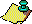Contents Next: A Sixteenth Order Up: Approximations to via the Previous: The Septic Iteration

# Nonic Iterations

In this section we explore nonic (ninth order) iterations. Of course, we may construct a ninth order iteration by iterating the cubic. We have found a nonic iteration which does not seem to come from the cubic.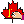Our nonic iteration is based on a symbolic discovery

that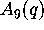is a nice eta-product: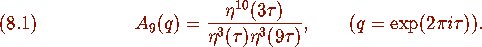This identity can be proved easily from results in Ramanujan's notebook. From (3.15), (3.16) we have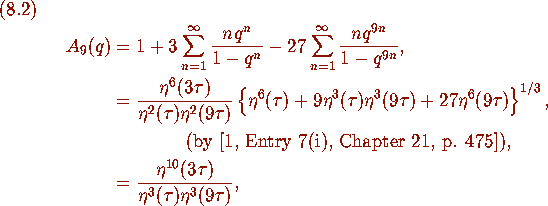by [1, Entry 1(iv), Chapter 20, p. 345,]. In the last step we have used the classical cubic modular equation for the eta-function: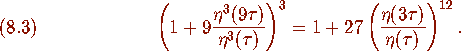See [7, Corollary 2.5,]. Using (6.5), (6.6)may be written terms of either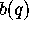or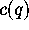(defined in (6.2), (6.3)), which were involved in the cubic iteration. We have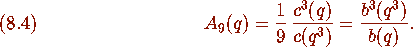This time we let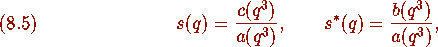where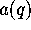,,are defined in (6.1)--(6.3). We define the following functions: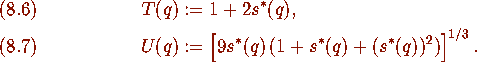Using the results of Section 6 it can be shown that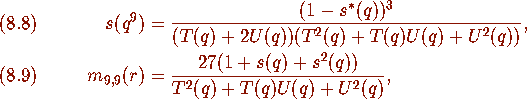where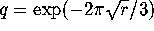. We can also utilize the initial values for the cubic and obtain a nonic iteration.

### Theorem 8.1

Define sequences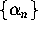,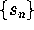, and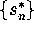by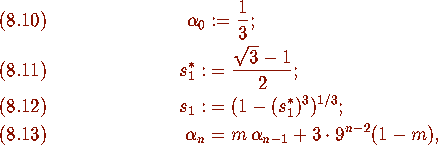where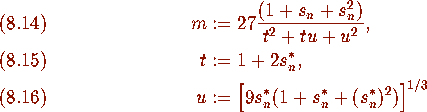and where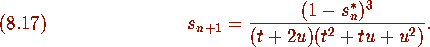Then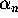converges nonically to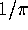.Contents Next: A Sixteenth Order Up: Approximations to via the Previous: The Septic Iteration